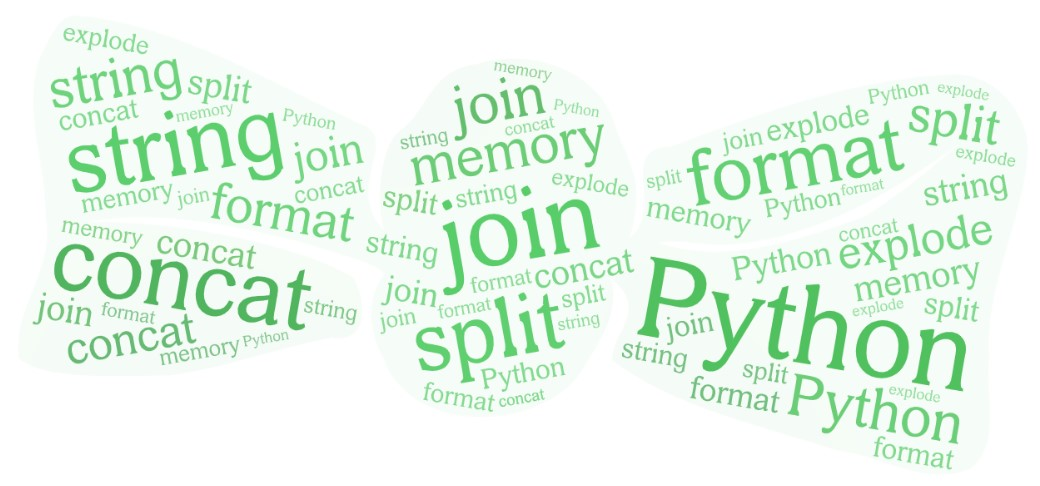# Python elementary string operationsLet’s start observing the Python strings.

Example:

``````s = 'string ☕'
print(type(s))
print(len(s))
``````

Output:

``````<class 'str'>
8
``````

If you create a simple string `s` you will get the class of string is str, and the length of string is 8 characters.

This doesn’t tell much. Let’s create several examples nailing it down what strings really are.

## The size of a single character

Example:

``````s  = 'a'
takes_bytes = sys.getsizeof(s+s)-sys.getsizeof(s)
print(takes_bytes)
print(ord(s))

s  = 'а'
takes_bytes = sys.getsizeof(s+s)-sys.getsizeof(s)
print(takes_bytes)
print(ord(s))
``````

Output:

``````1
97
2
1072
``````

What!?

Why there is a difference? Isn’t `a` the same as `а`?

They are not. The first a uses 1 byte per char, and the `ord` function returns the code point for a is 97. The second а code point is 1072.

Example:

``````str  = '👍'
takes_bytes = sys.getsizeof(str+str)-sys.getsizeof(str)
print(takes_bytes)
print(ord(str))
``````

Output:

``````4
128077
``````

In here the code point for the `👍` character is 128077

Python strings use three kind of chars: 1 byte char, 2 bytes char, and 4 bytes char.

## The size of the empty string

Example:

``````import sys
str  = ''
sys.getsizeof(str)
``````

Output:

``````51
``````

Example:

``````import sys
str  = ' '
sys.getsizeof(str)
``````

Output:

``````50
``````

What!?

Python empty strings takes more space than the simple space string. It’s true.

Python strings will take initially 50+ bytes to store information such as: length, length in bytes, hash, the encoding, and different string flags.

## Tricky interning

When working with short strings, python may internally memorize the same character under the same memory address. This is called string interning.

``````s = 'the example'
print(s)
print(s, s, s)
id(s), id(s), id(s)
``````

Output:

``````the example
e e e
(2172370743728, 2172340813616, 2172340813616)
``````

In here the characters e from the word “example” point to the same memory address. This saves memory.

Things get more evident in the next example: Example:

``````s = 'eee'
print(s)
print(s, s, s)
id(s), id(s), id(s)
``````

Output:

``````eee
e e e
2172340813616, 2172340813616, 2172340813616
``````

## String operations

### Concatenation

Let’s create a string of first 99 numbers:

Example:

``````s = ''
for x in range(1,100):
s=s+str(x)

print s
``````

Output:

``````123456789101112131415161718192021222324252627282930313233343536373839404142434445464748495051525354555657585960616263646566676869707172737475767778798081828384858687888990919293949596979899
``````

What we just did in here? We used the string concatenation operator `+`, and we converted each integer number from the range 1..100 into a string with the `str()` function.

Next we should use the `*` operator on strings.

Example:

``````'string'*3
``````

Output:

``````'stringstringstring'
``````

This would be again concatenation.

Note in Python strings do not have the `append()` method like in Java. In Python the `append` function works on lists.

### Splitting strings

Python `split()` is one of the finest splitting methods in the world. It works on characters, special characters or on words.

#### Splitting by character

Example:

``````txt = 'May the force be with you'
spl = txt.split('a')
print(spl)
``````

Output:

``````['M', 'y the force be with you']
``````

By default if you don’t provide any argument to `split()` it will split by any whitespace including regular space, non breaking space, new line, tabulator, etc.

Example:

``````txt = 'May the force be with you'
spl = txt.split()
print(spl)
``````

Output:

``````['May', 'the', 'force', 'be', 'with', 'you']
``````

#### Splitting by multiple characters

Example:

``````import re
res = re.split('[aeiou]', 'May the force be with you.')
print(res)
``````

Output:

``````['M', 'y th', ' f', 'rc', ' b', ' w', 'th y', '', '.']
``````

#### Splitting by word

Example:

``````txt = 'May the force be with you'
spl = txt.split('force')
print(spl)
``````

Output:

``````['May the ', ' be with you']
``````

#### Splitting using splitlines()

In some cases we need to split the text into lines first. For that we use `splitlines()`.

Example:

``````text='''file1.txt 2012 How to split text into lines?
file2.txt 2013 How do we stop splitting after several splits?
file3.txt 2020 Example maxsplit and splitlines'''

list =[]

for line in text.splitlines():
list.append(line.split(' ', maxsplit=2))

list
``````

Output:

``````[['file1.txt', '2012', 'How to split text with success?'],
['file2.txt', '2013', 'How do we stop splitting?'],
['file3.txt', '2020', 'Example of maxsplit and splitlines']]
``````

### Joining list elements to a string

Example:

``````lst = ['Join', 'list', 'elements', 'to', 'a', 'string']
s = ''.join(lst)
print(s)
``````

Output:

``````Joinlistelementstoastring
``````

That’s strange! With an additional improvement we will fix it. Example:

``````lst = ['Join', 'list', 'elements', 'to', 'a', 'string']
s = ' '.join(lst)
print(s)
``````

Output:

``````Join list elements to a string
``````

### String explosion to chars

In PHP there is `explode` method on strings. There is no such method in Python, instead you do the explosion like this:

Example:

``````lst = [x for x in 'explode']
print(lst)
``````

Output:

``````['e', 'x', 'p', 'l', 'o', 'd', 'e']
``````

### Reverse string

Programming tutorials usually have examples on how to reverse a string. This is easy in Python:

Example:

``````str = 'reverse'
str = str[::-1]
print(str)
``````

Output:

``````esrever
``````

### String replace

The easy way would be to use `str.replace()`.

Example:

``````str = 'eldorada'
print(str)

str = str.replace('da', 'do')
print(str)
``````

Output:

``````eldorada
``````

Note that string replace operation is irreversible.

The other way would be to use the `re` regular expressions.

Example:

``````import re
s = "Exaample String"
print(s)
s = re.sub(r'a+', r'a', s)
print(s)
``````

Output:

``````Exaample String
Example String
``````

## Appendix : String Methods

capitalize casefold center
count encode endswith
expandtabs find format
format_map index isalnum
isalpha isascii isdecimal
isdigit isidentifier islower
isnumeric isprintable isspace
istitle isupper join
ljust lower lstrip
maketrans partition replace
rfind rindex rjust
rpartition rsplit rstrip
split splitlines startswith
strip swapcase title
translate upper zfill

## String literals notation

You can use both of the notations using single quotes `''` or double quotes `""`.

However, for printing Python uses single quotes.

Example:

``````l= ["string" , 'string']
print(l)
``````

Output:

``````['string', 'string']
``````

tags: string & category: python